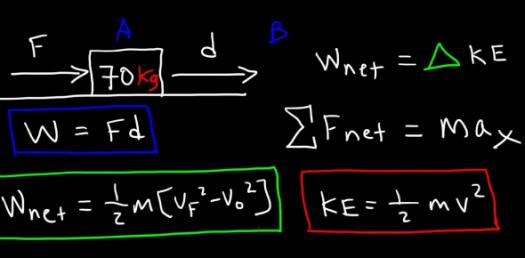# Work And Energy Questions! Physics Trivia Quiz

5 Questions | Attempts: 89Settings.

• 1.
Which of the following sentences uses work in the scientific sense?
• A.

Stan goes to work on the bus.

• B.

Anne did work on the project for 5 hours.

• C.

Joseph found that holding the banner in place was hard work.

• D.

An engine does work on a car when the car is moving.

• 2.
A painter lifts a bucket of paint, carries it 5 m horizontally, then sets it back down. Which of the following is true?
• A.

The force of gravity does negative work when the worker lifts the bucket.

• B.

The painter does positive work on the bucket when carrying it horizontally at a constant speed.

• C.

The painter does positive work on the bucket when setting it down.

• D.

No net work is done on the bucket.

• 3.
Which equation is used to calculate the work done on an object by a force at an angle, q, to the displacement?
• A.

W = Fd

• B.

W = Fdcos theta

• C.

W = Fdsin theta

• D.

W = mgsin theta

• 4.
A joule is equivalent to a
• A.

N.

• B.

N•m.

• C.

N/m.

• D.

Kg•m/s2.

• 5.
A parachutist falls at a constant speed for 200 m. Which of the following is true?
• A.

The force of gravity is the only force doing work on the parachutist.

• B.

Air resistance is the only force doing work on the parachutist.

• C.

No forces are doing work on the parachutist.

• D.

No network is done on the parachutist.

## Related TopicsBack to top
×

Wait!
Here's an interesting quiz for you.